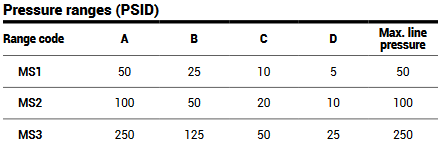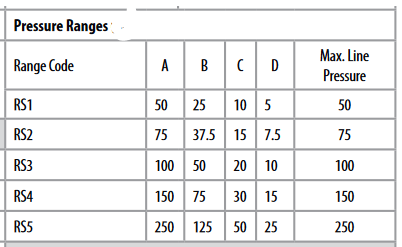# What is the max line pressure of the 231?

The max differential pressure you can measure is the max line pressure the unit can see. This is independent of the range code A through D you select.

For example:

• the Model 231GMS2 has a max differential pressure of 100PSID, so the max line pressure is 100PSI.
• The Model 231GMS1 has a max differential pressure of 50PSID, so the max line pressure is 50PSI
• If you select range code D (5PSI) on an MS1, the max line pressure is still the max differential pressure of 50PSI.